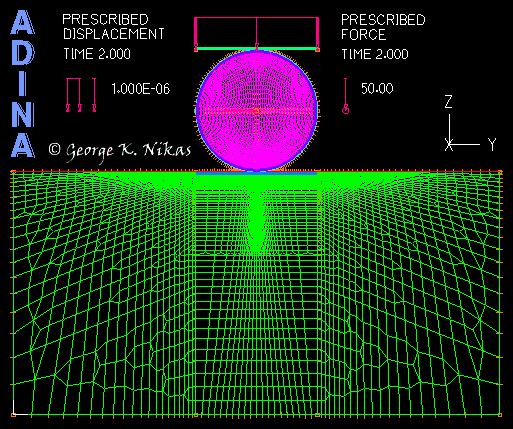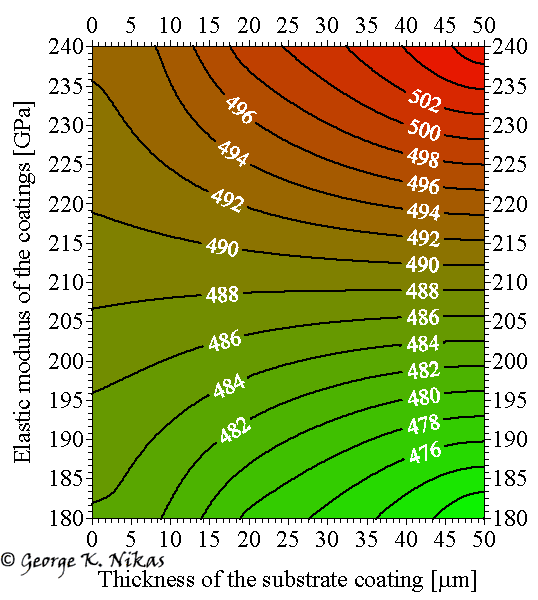Paper: Finite-element analysis of layered rolling contacts.

Authors: Nikas, G. K., Sayles, R. S.

Abstract

A fundamental, two-dimensional finite element analysis of coated surfaces in rolling contact is presented with detailed listing of all modelling steps. A large number of basic results from a parametric study has been derived, focusing on the stress analysis and the effect of coatings on the life expectancy of coated surfaces, without actually getting into the ambiguities of fatigue life evaluation. Parameters included in the study are the metallic coating thickness (from zero to 50 µm) and elastic modulus (from 180 to 240 GPa), the Coulomb friction coefficient (zero, 0.05 and 0.10) and the endurance limit of the coated substrate. A generalisation involves the application of traction to simulate driving or driven wheels. The effect of that traction on the stress results is thoroughly examined. The set of results, summarized in a figure with nine diagrams, assists the quick selection of the optimum parameter values for maximising coating performance and minimising the risk of contact fatigue within the design limits used in the study. The model is realistic in that it does not use idealised load distributions in the rolling contact but actual geometrical solids in contact under normal operating conditions, letting the finite element analysis software resolve the loads and stresses, which are subsequently validated.

Some figures from this work

Figure 1 shows the finite element mesh for the rolling contact of a coated cylinder on a coated substrate in plane strain (two-dimensional analysis). The mesh is intelligently constructed to be dense in the areas of interest and coarse in areas where accuracy is of less importance, such that computational speed is not compromised. This model contains approximately 21,000 to 33,000 solid, 9-node elements. The minimum element size in the coatings is equal to about 1 µm. A prescribed, downward vertical displacement is applied to a rigid line on the top of the roller to compress the roller on to the substrate. The vertical displacement is followed by the application of a uniformly distributed, horizontal force F on the axis of the roller. The horizontal force introduces tangential loading to the roller-substrate contact, emulating rolling conditions in this otherwise static contact. The arrangement gives effective rolling friction coefficients between zero and 0.1. Dry and lubricated contact conditions are emulated this way. The model was developed with the commercial software ADINA and was validated using established contact mechanics theories, showing excellent agreement, as detailed in the paper.Fig. 1. Finite element mesh for the 2-d rolling contact of a coated cylinder on a coated substrate.

Figure 2 shows the effect of the substrate coating thickness and the elastic modulus of both coatings on the maximum absolute normal z-stress (see contour lines with labels in MPa) for F = 150 N, assuming that the Coulomb friction coefficient between the contacting coatings is equal to 0.05. The vertical displacement of the roller is equal to 1 µm.Fig. 2. Effect of the substrate coating thickness and coatings elastic modulus on the maximum absolute normal z-stress (see contour lines with labels in MPa) for F = 150 N, assuming a Coulomb friction coefficient between the contacting coatings equal to 0.05.

The paper contains a total of 60 performance diagrams. The results are summarized in a figure with nine diagrams, which facilitates the selection of the optimum parameter values for maximising coating performance and minimising the risk of contact fatigue within the design limits used in the study.

Homepage of Dr Nikas